# PartialLineForce

Base model for massless line force elements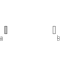# Information

This information is part of the Modelica Standard Library maintained by the Modelica Association.

All massless line force elements should be based on this base model. This model defines frame_a and frame_b, computes the (guarded) relative distance s and provides the force and torque balance of the cut-forces and cut-torques at frame_a and frame_b, respectively. In sub-models, only the line force f, acting at frame_b on the line from frame_a to frame_b, as a function of the relative distance s and its derivative der(s) has to be defined. Example:

```   model Spring
parameter Real c "spring constant",
parameter Real s_unstretched "unstretched spring length";
extends Modelica.Mechanics.MultiBody.Interfaces.PartialLineForce;
equation
f = c*(s-s_unstretched);
end Spring;
```

# Parameters (3)

s_small Value: 1e-10 Type: Distance (m) Description: Prevent zero-division if distance between frame_a and frame_b is zero Value: false Type: Boolean Description: =true, if rotation frame_a.R is fixed (to directly connect line forces) Value: false Type: Boolean Description: =true, if rotation frame_b.R is fixed (to directly connect line forces)

# Connectors (2)

frame_a frame_b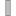Type: Frame_a Description: Coordinate system a fixed to the component with one cut-force and cut-torque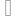Type: Frame_b Description: Coordinate system b fixed to the component with one cut-force and cut-torque

# Components (1)

 world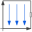Type: World

# Extended by (3)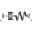SpringDamperSeries Modelica.Mechanics.MultiBody.Forces Linear spring and linear damper in series connection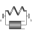SpringDamperParallel Modelica.Mechanics.MultiBody.Forces Linear spring and linear damper in parallel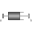Damper Modelica.Mechanics.MultiBody.Forces Linear (velocity dependent) damper